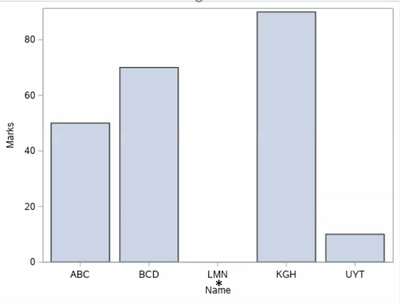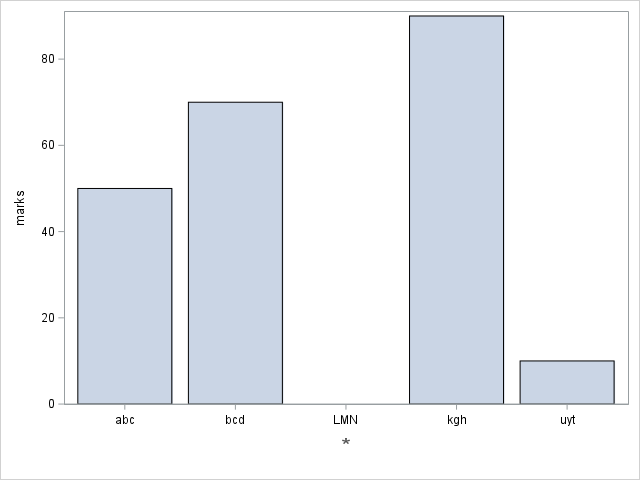## Bar plot, To present symbol (Star, Plus sign) on X-axis For specific bars (separate variable)

I am having simple Bar plot. See below code. In SCORE dataset for name "LMN" doesn't have any marks. I want to present star(*) at the x-axis below tickvalues (I can hide tickvalues).

Any thoughts How to present Star(*) at axis, indicating it is different from dataset row having no values.

data score;

input name \$1-3 marks 5.;

datalines;

abc 50

bcd 70

LMN

kgh 90

uyt 10

;

run;

proc template;

define statgraph temp;

begingraph;

layout overlay;

barchart x=name y=score;

endlayout;

endgraph;

end;

run;

proc sgrender data=score template=temp;

run;1 ACCEPTED SOLUTION

Accepted Solutions

## Re: Bar plot, To present symbol (Star, Plus sign) on X-axis For specific bars (separate variable)

You can use the XAXISTABLE statement for this. Why not simply use Proc SGPLOT for the graph. See example below

``````data score;
infile cards dlm="," dsd missover;
input name \$ marks ;
if missing(marks) then do;
marker = "*";
end;
datalines;
ABC,50
BCD,
LMN,
KGH,90
UYT,10
;

proc sgplot data=score tmplout="c:\temp\sgplot2gtl.sas";
vbarbasic name / response=marks;
xaxistable marker / nolabel location=inside valueattrs=(size=15pt color=red) ;  xaxistable marker / nolabel location=outside valueattrs=(size=15pt color=red) ;
run;``````

2 REPLIES 2

## Re: Bar plot, To present symbol (Star, Plus sign) on X-axis For specific bars (separate variable)

You can use the XAXISTABLE statement for this. Why not simply use Proc SGPLOT for the graph. See example below

``````data score;
infile cards dlm="," dsd missover;
input name \$ marks ;
if missing(marks) then do;
marker = "*";
end;
datalines;
ABC,50
BCD,
LMN,
KGH,90
UYT,10
;

proc sgplot data=score tmplout="c:\temp\sgplot2gtl.sas";
vbarbasic name / response=marks;
xaxistable marker / nolabel location=inside valueattrs=(size=15pt color=red) ;  xaxistable marker / nolabel location=outside valueattrs=(size=15pt color=red) ;
run;``````

## Re: Bar plot, To present symbol (Star, Plus sign) on X-axis For specific bars (separate variable)

Another way would be to annotate the * character:

data score;
input name \$1-3 marks 5.;
datalines;
abc 50
bcd 70
LMN
kgh 90
uyt 10
;
run;

data score_anno; set score (where=(marks=.));
length label \$300 anchor x1space y1space function textcolor \$50;
layer='front';
x1space='datavalue'; xc1=name;
y1space='graphpercent'; y1=7;
function='text'; label='*';
textcolor='gray55'; textsize=15;
width=100; widthunit='percent'; anchor='center';
run;